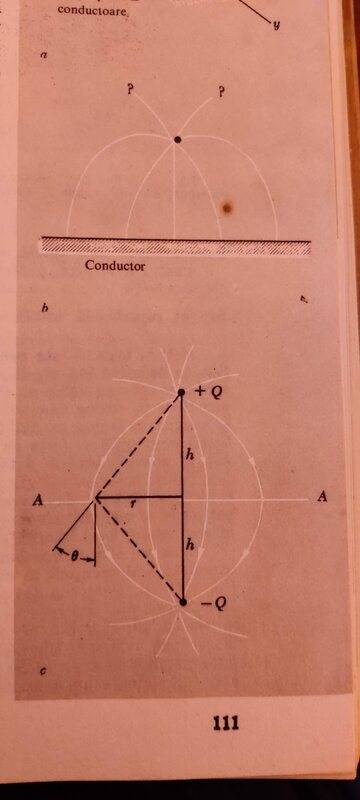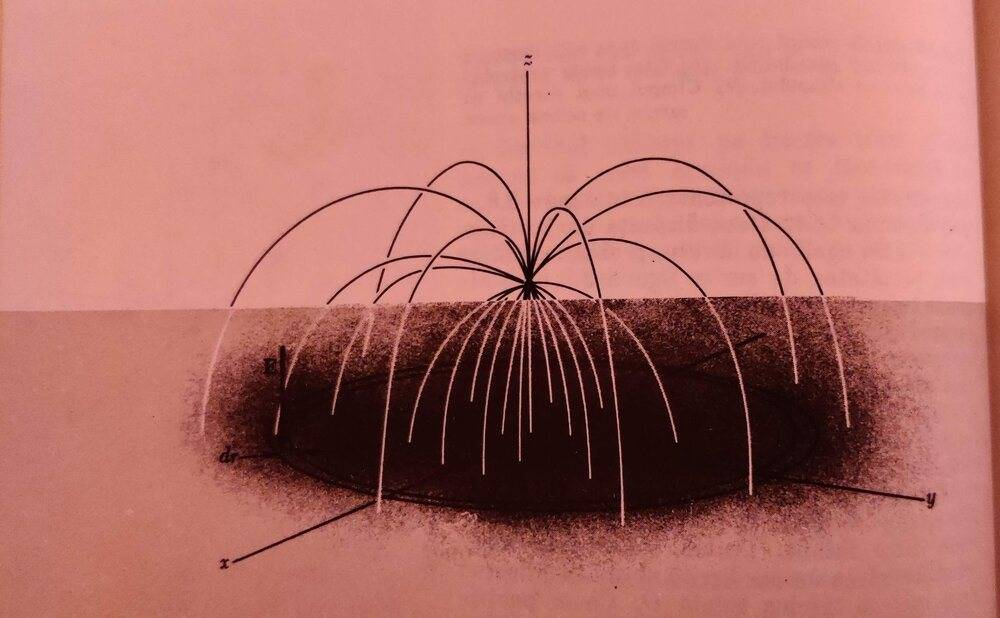# Electric field lines between a point-charge and a conducting sheet

huszarerik
Homework Statement:
In the electric field of the point-charge (Q) above the conducting sheet (fig 1), we follow an electric field line, which leaves the point-charge parallel to the sheet. Where will the electric field line meet the surface of the conducting sheet?
Relevant Equations:
Gauss's Law: ∫ E⋅da=Qin/ε
Charge density on the conductor: σ=-Q*h/2*π*(r^2+h^2)^(3/2)
where:
h = distance from the point-charge to the sheet
r = distance along the horizontal axis on the conducting sheetfigure 1: →I don't understand how to approach this problem. Basically it asks for the distance r.I think I should use Gauss's law, but I've been thinking about the shape of the gaussian surface and I'm not sure about how it should look or where I should place it. Any help would be useful, especially in terms of ideas.
Thank you.

Last edited: